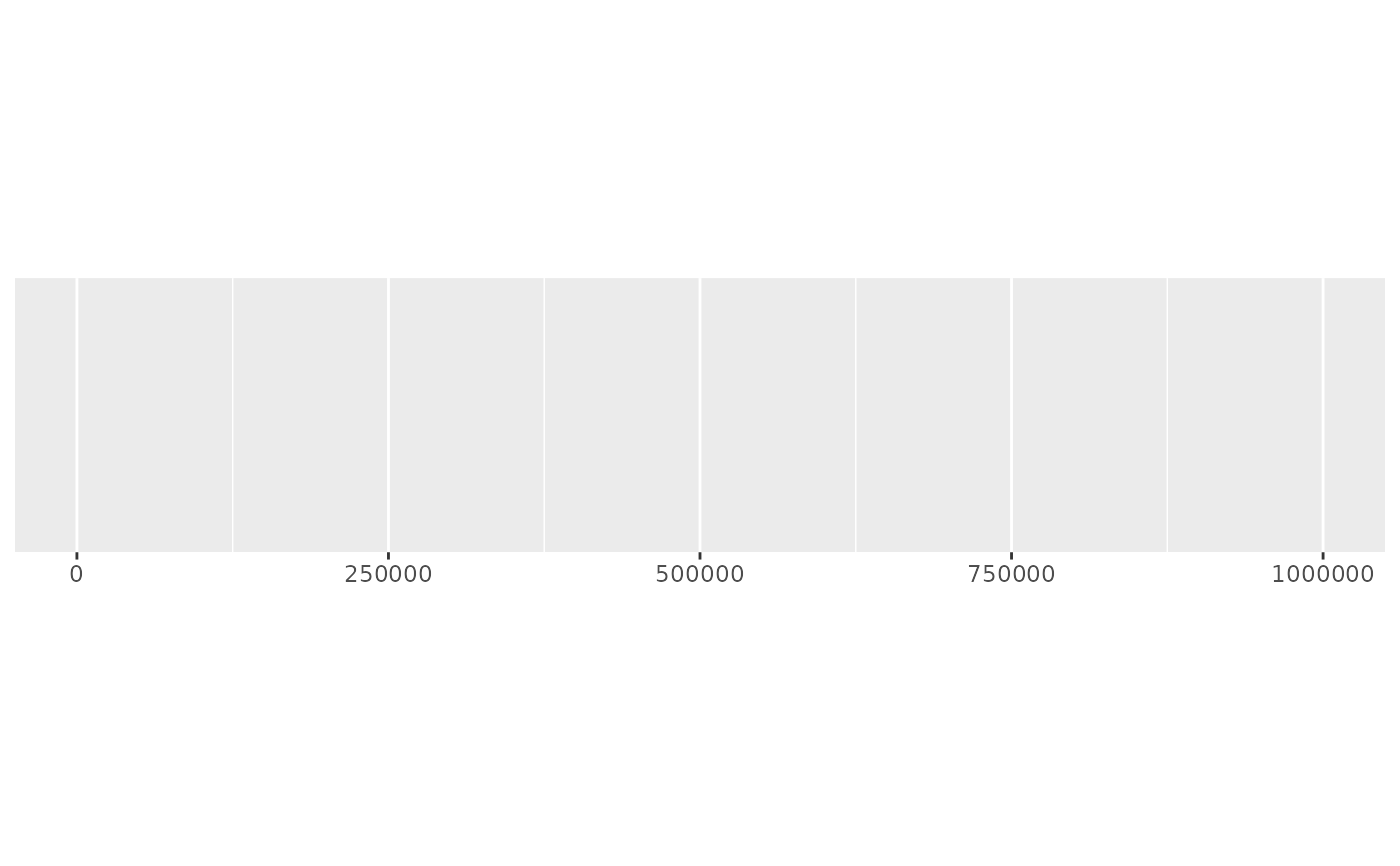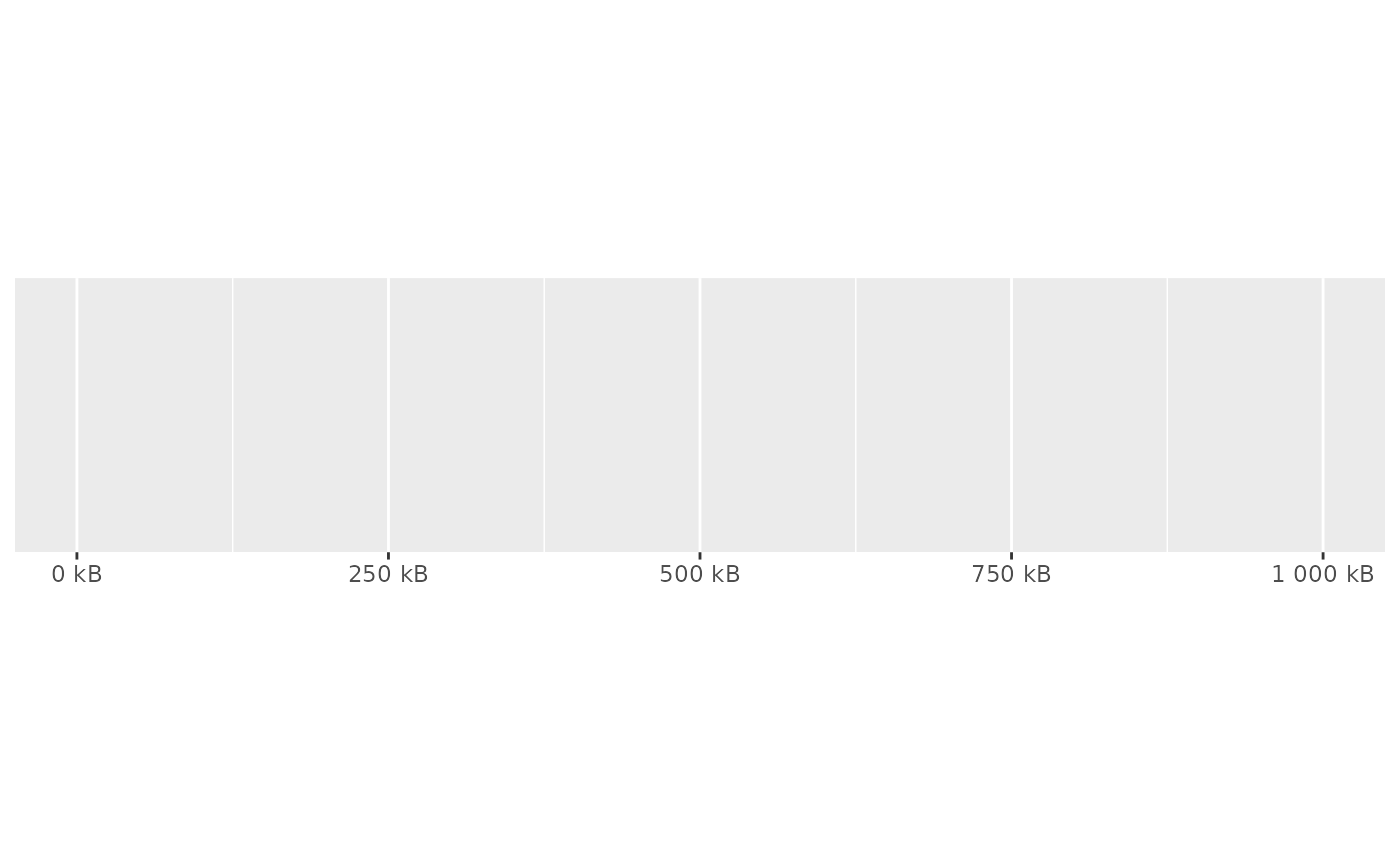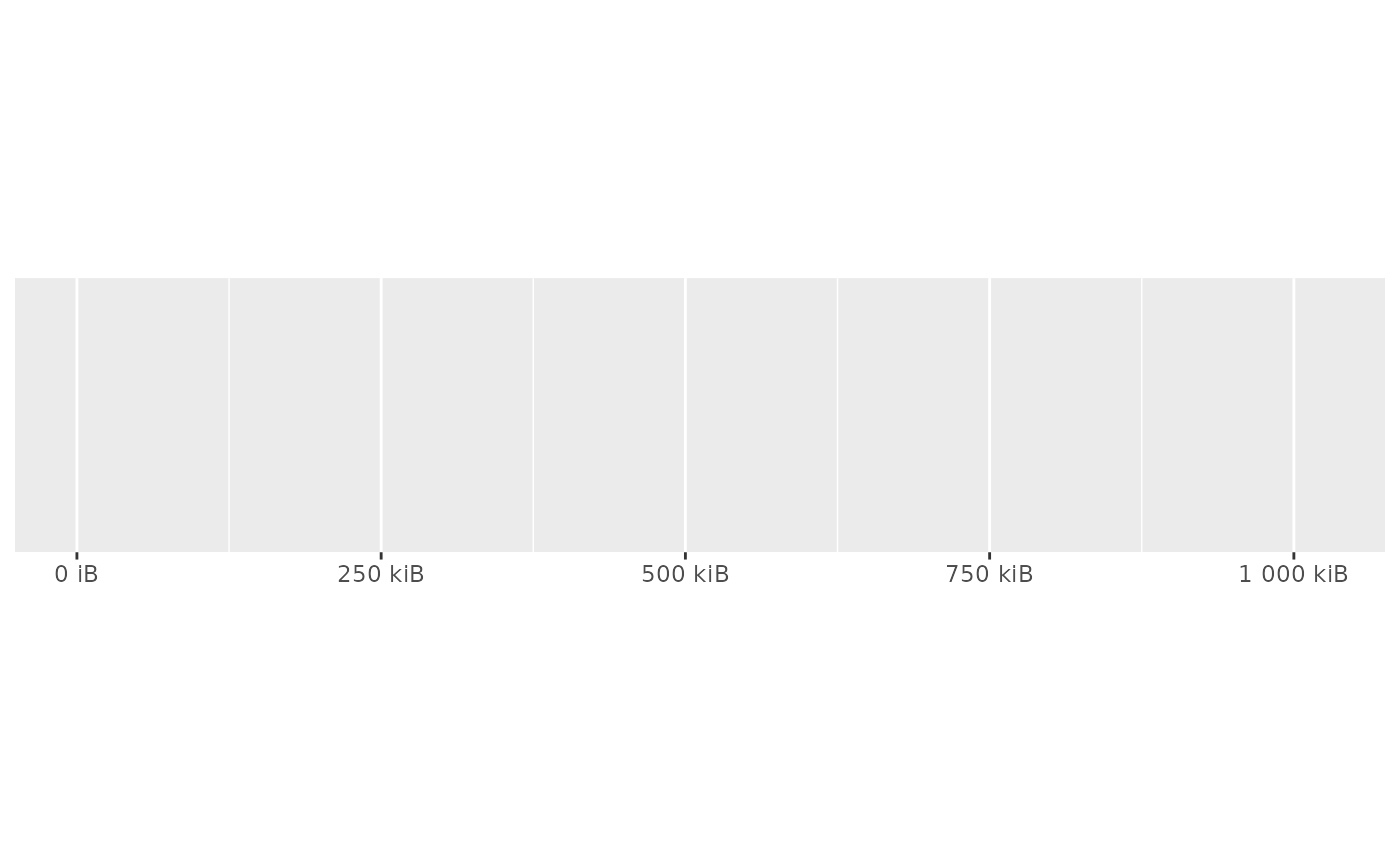Scale bytes into human friendly units. Can use either SI units (e.g. kB = 1000 bytes) or binary units (e.g. kiB = 1024 bytes). See Units of Information on Wikipedia for more details.

## Usage

label_bytes(units = "auto_si", accuracy = 1, scale = 1, ...)

## Arguments

units

Unit to use. Should either one of:

• "kB", "MB", "GB", "TB", "PB", "EB", "ZB", and "YB" for SI units (base 1000).

• "kiB", "MiB", "GiB", "TiB", "PiB", "EiB", "ZiB", and "YiB" for binary units (base 1024).

• auto_si or auto_binary to automatically pick the most appropriate unit for each value.

accuracy

A number to round to. Use (e.g.) 0.01 to show 2 decimal places of precision. If NULL, the default, uses a heuristic that should ensure breaks have the minimum number of digits needed to show the difference between adjacent values.

Applied to rescaled data.

scale

A scaling factor: x will be multiplied by scale before formatting. This is useful if the underlying data is very small or very large.

...

Other arguments passed on to number()

## Value

A labeller function that takes a numeric vector of breaks and returns a character vector of labels.

Other labels for continuous scales: label_dollar(), label_number_auto(), label_number_si(), label_ordinal(), label_parse(), label_percent(), label_pvalue(), label_scientific()

Other labels for log scales: label_log(), label_number_si(), label_scientific()

## Examples

demo_continuous(c(1, 1e6))
#> scale_x_continuous()demo_continuous(c(1, 1e6), labels = label_bytes())
#> scale_x_continuous(labels = label_bytes())# Auto units are particularly nice on log scales
demo_log10(c(1, 1e7), labels = label_bytes())
#> scale_x_log10(labels = label_bytes())# You can also set the units
demo_continuous(c(1, 1e6), labels = label_bytes("kB"))
#> scale_x_continuous(labels = label_bytes("kB"))# You can also use binary units where a megabyte is defined as
# (1024) ^ 2 bytes rather than (1000) ^ 2. You'll need to override